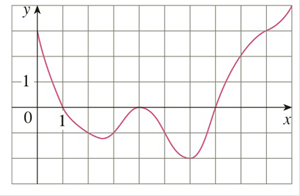Chapter 4.2, Problem 5E

Chapter
Section
Textbook Problem

# The graph of a function f is given. Estimate ∫ 0 10 f ( x ) d x using five subintervals with (a) right endpoints, (b) left endpoints, and (c) midpoints.To determine

Part (a):

To estimate:

010fxdx  using five subintervals with right end points.

Explanation

1) Concept:

Use theorem (4) to estimate the value of the integral by using the right end points.

2) Theorem (4):

If f is integrable on [a, b] then

abf(x)dx=limni=1nf(xi)x

where  x=b - an,n is the number of the subintervals and xi=a+ix

Then an estimate of abf(x)dx using 5 subintervals and right end points is given by

i=15f(xi)x

Where x=b - a5 and xi are right end points of corresponding intervals

3) Given:

The graph:

4) Calculation:

Here, n=5, a=0 and b=10

So, the width of the subintervals is

x=b-an

x=10-05

x=105=2

By using width  x=2 form the subintervals:

0,2, 2, 4, 4, 6, 6, 8 and [8, 10]

So, the right endpoints are x1=2,

To determine

Part (b):

To estimate:

010fxdx  using five subintervals with the left end points.

To determine

Part (c):

To estimate:

010fxdx  using five subintervals with midpoints

### Still sussing out bartleby?

Check out a sample textbook solution.

See a sample solution

#### The Solution to Your Study Problems

Bartleby provides explanations to thousands of textbook problems written by our experts, many with advanced degrees!

Get Started

#### Solve the equations in Exercises 126. x4x+1xx1=0

Finite Mathematics and Applied Calculus (MindTap Course List)

#### In Exercises 13-20, sketch a set of coordinate axes and plot each point. 18. (52,32)

Applied Calculus for the Managerial, Life, and Social Sciences: A Brief Approach

#### Solve for x: e2x1 = 10. a) 12(1+10e) b) 3 + ln 10 c) 2 + ln 10 d) 1+ln102

Study Guide for Stewart's Single Variable Calculus: Early Transcendentals, 8th